# 3D 碰撞检测

## 轴对齐包围盒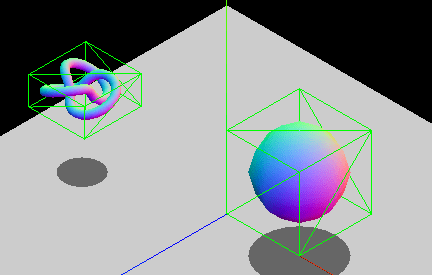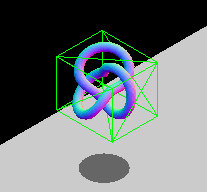### 点与 AABB

$f\left(P, B\right)= \left(P_x \ge B_\left\{minX\right\} \wedge P_x \le B_\left\{maxX\right\}\right) \wedge \left(P_y \ge B_\left\{minY\right\} \wedge P_y \le B_\left\{maxY\right\}\right) \wedge \left(P_z \ge B_\left\{minZ\right\} \wedge P_z \le B_\left\{maxZ\right\}\right)$

js

function isPointInsideAABB(point, box) {
return (
point.x >= box.minX &&
point.x <= box.maxX &&
point.y >= box.minY &&
point.y <= box.maxY &&
point.z >= box.minZ &&
point.z <= box.maxZ
);
}


### AABB 与 AABB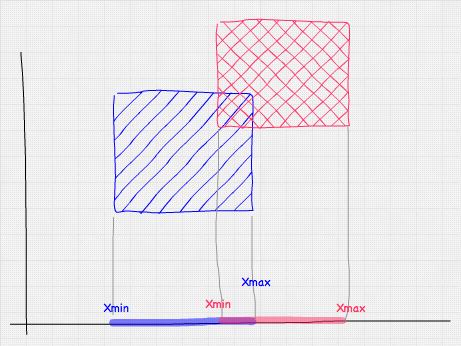$f\left(A, B\right) = \left(A_\left\{minX\right\} \le B_\left\{maxX\right\} \wedge A_\left\{maxX\right\} \ge B_\left\{minX\right\}\right) \wedge \left( A_\left\{minY\right\} \le B_\left\{maxY\right\} \wedge A_\left\{maxY\right\} \ge B_\left\{minY\right\}\right) \wedge \left(A_\left\{minZ\right\} \le B_\left\{maxZ\right\} \wedge A_\left\{maxZ\right\} \ge B_\left\{minZ\right\}\right)$

js

function intersect(a, b) {
return (
a.minX <= b.maxX &&
a.maxX >= b.minX &&
a.minY <= b.maxY &&
a.maxY >= b.minY &&
a.minZ <= b.maxZ &&
a.maxZ >= b.minZ
);
}


## 包围球

### 点与球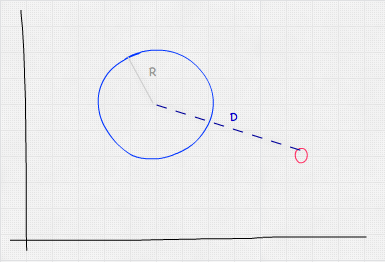$f\left(P,S\right) = S_\left\{radius\right\} \ge \sqrt\left\{\left(P_x - S_x\right)^2 + \left(P_y - S_y\right)^2 + \left(P_z - S_z\right)^2\right\}$

js

function isPointInsideSphere(point, sphere) {
// 我们使用乘法是因为这样比调用 Math.pow 更快
const distance = Math.sqrt(
(point.x - sphere.x) * (point.x - sphere.x) +
(point.y - sphere.y) * (point.y - sphere.y) +
(point.z - sphere.z) * (point.z - sphere.z),
);
}


### 球体与球体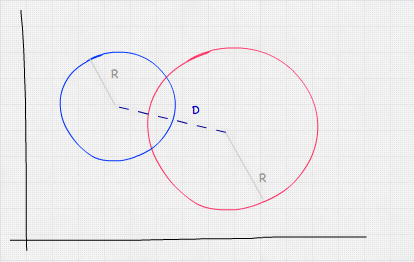$f\left(A,B\right) = \sqrt\left\{\left(A_x - B_x\right)^2 + \left(A_y - B_y\right)^2 + \left(A_z - B_z\right)^2\right\} \le A_\left\{radius\right\} + B_\left\{radius\right\}$

js

function intersect(sphere, other) {
// 我们使用乘法是因为这样比调用 Math.pow 更快
const distance = Math.sqrt(
(sphere.x - other.x) * (sphere.x - other.x) +
(sphere.y - other.y) * (sphere.y - other.y) +
(sphere.z - other.z) * (sphere.z - other.z),
);
}


### 球体与 AABB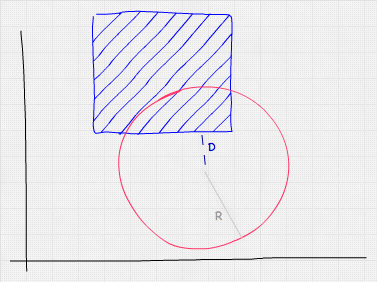js

function intersect(sphere, box) {
// 通过逼近距离获得距离球体中心最近的点
const x = Math.max(box.minX, Math.min(sphere.x, box.maxX));
const y = Math.max(box.minY, Math.min(sphere.y, box.maxY));
const z = Math.max(box.minZ, Math.min(sphere.z, box.maxZ));

// 这与 isPointInsideSphere 相同
const distance = Math.sqrt(
(x - sphere.x) * (x - sphere.x) +
(y - sphere.y) * (y - sphere.y) +
(z - sphere.z) * (z - sphere.z),
);

}


## 使用物理引擎

3D 物理引擎提供了碰撞检测算法，其中大多数也都是基于包围体。物理引擎的工作方式是创建一个物理体，通常附加到其可视化表示上。该物体具有诸如速度、位置、旋转、扭矩等属性，还有一个物理形状。这个形状是碰撞检测计算中考虑的形状。

MDN 上的相关文章：